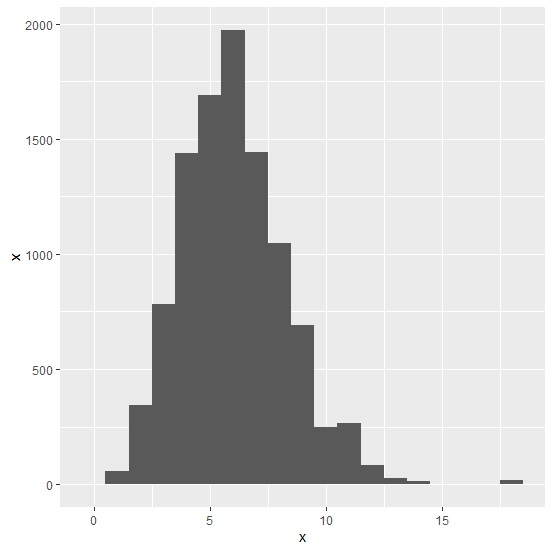# How to create histogram for discrete column in an R data frame?

R ProgrammingServer Side ProgrammingProgramming

To create histogram for discrete column in an R data frame, we can use geom_bar function of ggplot2 package and set the width to 1 also passing same column for x and y in aes.

For example, if we have a data frame called df that contains a discrete column say x then the histogram for data in x can be created by using the below given command −

ggplot(df,aes(x,x))+geom_bar(stat="identity",width=1)

## Example

Following snippet creates a sample data frame −

x<-rpois(2000,5)
df<-data.frame(x)
head(df,20)

## Output

The following dataframe is created −

   x
1  8
2  4
3  3
4  2
5  5
6  6
7  4
8  5
9  7
10 4
11 1
12 5
13 6
14 8
15 4
16 3
17 1
18 5
19 6
20 4

Now, to load the ggplot2 package and create histogram for data in x, add the following code to the above snippet −

library(ggplot2) ggplot(df,aes(x,x))+geom_bar(stat="identity",width=1)


## Output

If you execute all the above given snippets as a single program, it generates the following Output −